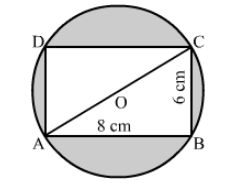# Find the area of the shaded region in the given figure,`
Question:

Find the area of the shaded region in the given figure, if ABCD is a rectangle with sides 8 cm and 6 cm and O is the centre of the circle.Solution:

In right triangle ABC
AC2 = AB2 + BC2
= 82 +62
= 64 + 36
= 100
∴ AC2 = 100
⇒ AC = 10 cm

Now, Radius of circle $(\mathrm{OA})=\frac{1}{2} \mathrm{AC}=5 \mathrm{~cm}$

Area of the shaded region = Area of circle − Area of rectangle OABC

$=\pi(\mathrm{OA})^{2}-\mathrm{AB} \times \mathrm{BC}$

$=\frac{22}{7} \times(5)^{2}-8 \times 6$

$=78.57-48$

$=30.57 \mathrm{~cm}^{2}$

Hence, the area of the shaded region is 30.57 cm2.